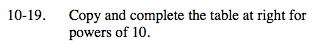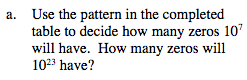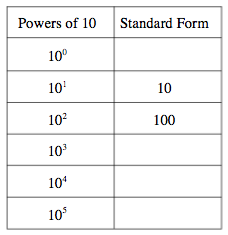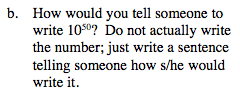### Home > MC2 > Chapter 10 > Lesson 10.1.2 > Problem10-19

10-19.Look at 101 = 10 and 102 = 100 closely. What do the exponents tell you about the standard form?

The exponent tells you how many zeros there are in the standard form. Complete the table. How many zeros will 1023 have?Look at the pattern in part (a) and describe how you would find 1050?